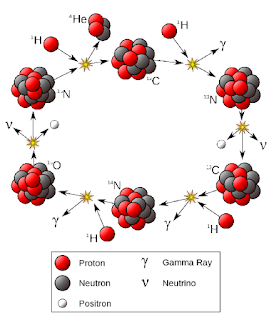### Section 2.2 - Stellar Thermonuclear Fusion

#### The Sun

The Sun is 4.6 billion years old and will continue to shine as is for another 5 billion years. What is it made of, and how does it power itself?

The Sun, like all other stars, powers itself through the thermonuclear fusion of hydrogen into helium. Under normal conditions, hydrogen nuclei don't spontaneously fuse into helium, but the pressure and temperature at the core of the Sun is so great that the nuclei fuse together through one of two primary processes (see below)

The Sun naturally wants to collapse in on itself due to its own gravity, but the outward pressure from the nuclear reaction balances the gravity into an equilibrium. As long as the Sun still has hydrogen to burn, this reaction will continue and the Sun will remain stable

#### The Proton-Proton Chain ReactionCredit: Wikipedia
Recalling that hydrogen contains one proton and helium contains two, let's break down the above diagram:

1. To start the chain reaction, two protons fuse, but one of them spontaneously decays into a neutron, emitting a positron, which immediately annihilates with a free electron floating in the Sun, producing a photon, and a neutrino, which flies out unimpeded. This is by far the hardest step to accomplish in the chain and the one that sets it off
2. Another proton then fuses, forming Helium-3 (two protons, one neutron)
3. Two Helium-3 nuclei fuse into a Helium-4 nucleus (two protons, two neutrons), the most common form of helium
4. Two protons are ejected, continuing the cycle

This process is more dominant in smaller, younger stars like the Sun

#### The CNO Cycle (Carbon - Nitrogen - Oxygen)Credit: Wikipedia

Over the course of various points in the cycle, 4 protons will fuse using carbon, nitrogen, and oxygen isotopes as catalysts to produce one helium nucleus, two positrons, and two neutrinos

$Step \thinspace 1: \quad {}^{12}_6C + {}^{1}_1H \rightarrow {}^{13}_7N + \gamma$

$Step \thinspace 2: \hspace{3.5em}^{13}_7N \rightarrow {}^{13}_6C + {}^{0}_{+1}e + v$

$Step \thinspace 3: \quad {}^{13}_6C + {}^{1}_1H \rightarrow {}^{14}_7N + \gamma$

$Step \thinspace 4: \quad {}^{14}_7N + {}^{1}_1H \rightarrow {}^{15}_8O + \gamma$

$Step \thinspace 5: \hspace{3.5em}^{15}_8O \rightarrow {}^{15}_7N + {}^{0}_{+1}e + v$

$Step \thinspace 6: \quad {}^{15}_7N + {}^{1}_1H \rightarrow {}^{12}_6O + {}^{4}_2He$

Like the proton-proton cycle, the positrons will annihilate with electrons to produce protons and the neutrinos will fly away. The Carbon-12 is present at both the beginning and the end, continuing the cycle

This process is more dominant in larger, order stars